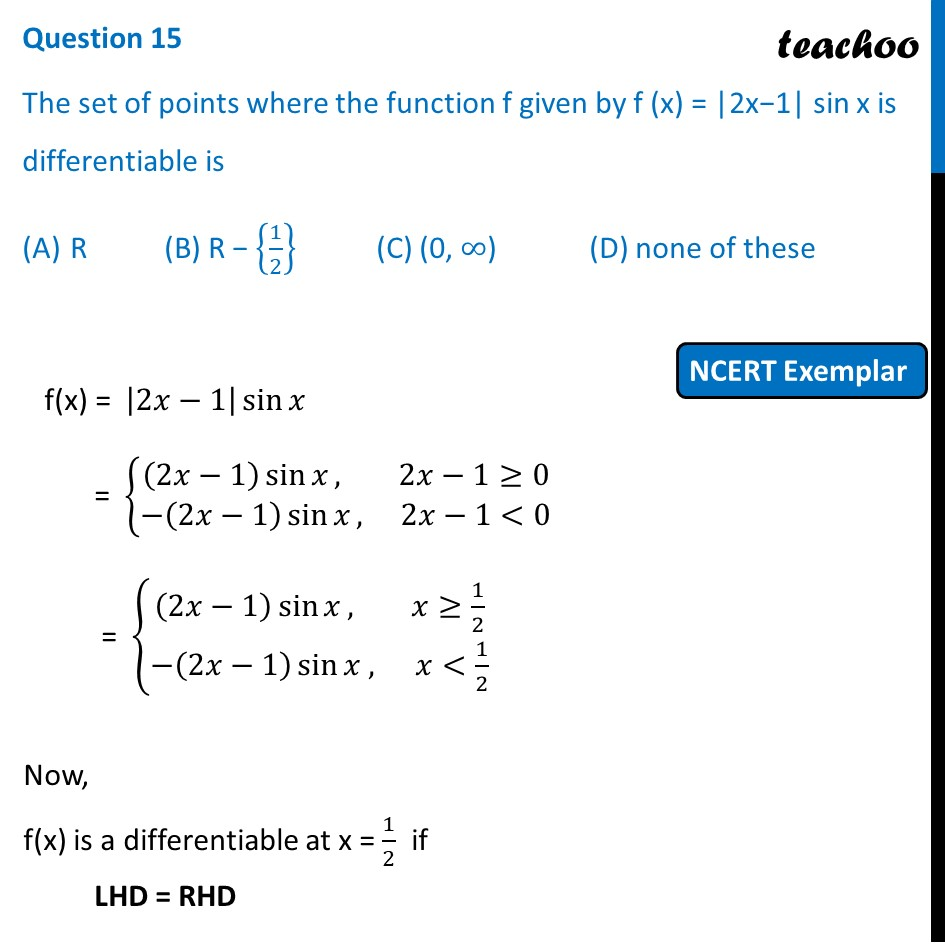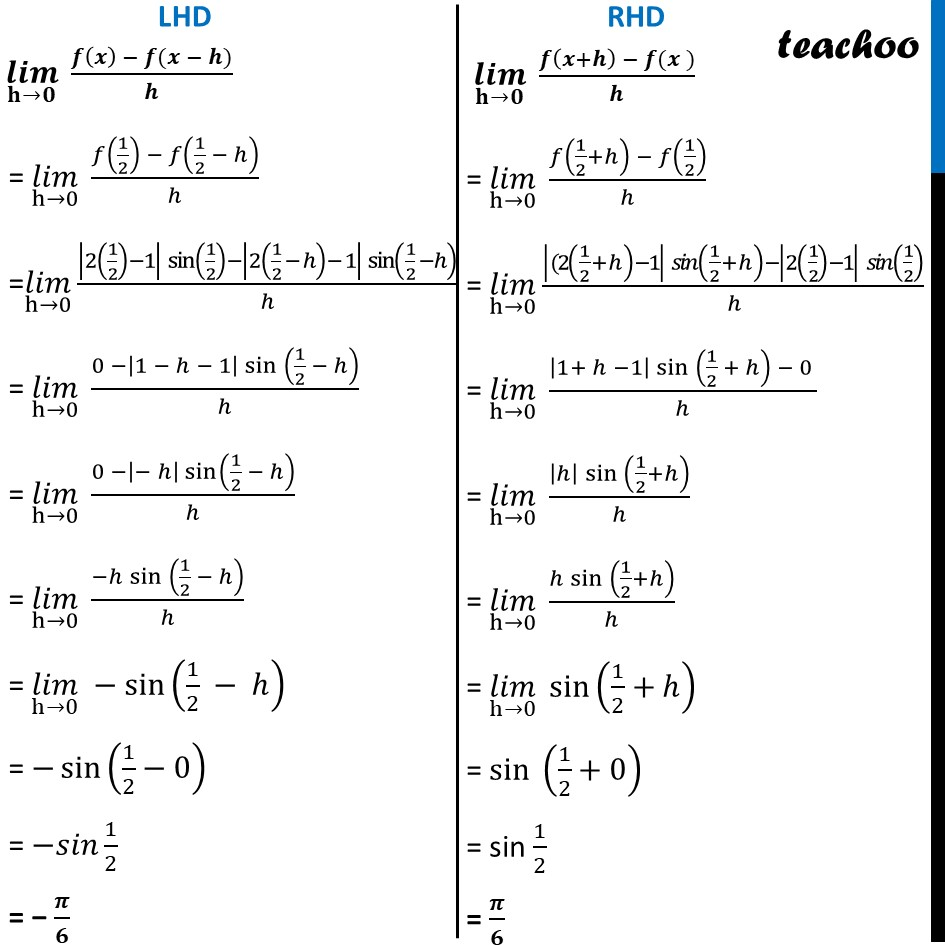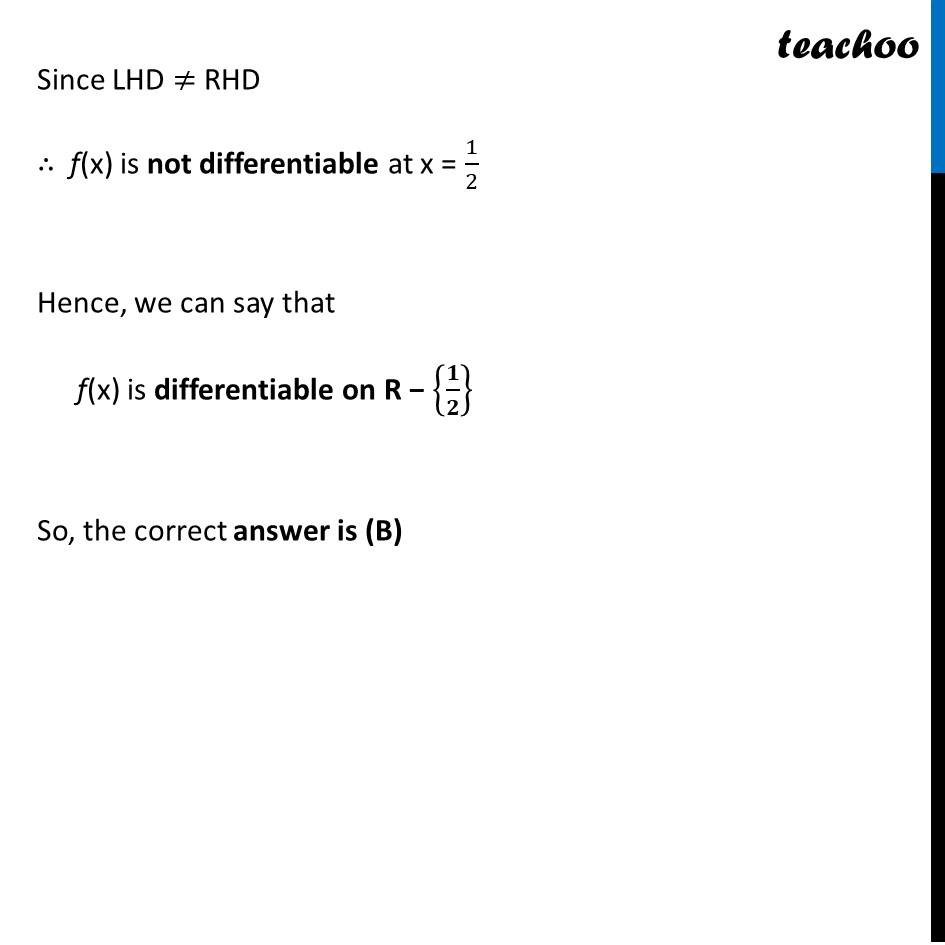NCERT Exemplar - MCQs

Chapter 5 Class 12 Continuity and Differentiability
Serial order wise

## (D) none of these

This question is similar to Ex 5.2, 9 - Chapter 5 Class 12 - Continuity and DifferentiabilityLearn in your speed, with individual attention - Teachoo Maths 1-on-1 Class

### Transcript

Question 13 The set of points where the function f given by f (x) = |2x−1| sin x is differentiable is R (B) R − {1/2} (C) (0, ∞) (D) none of these f(x) = |2𝑥−1| sin⁡𝑥 = {█((2𝑥−1) sin⁡𝑥, 2𝑥−1≥0@−(2𝑥−1) sin⁡𝑥, 2𝑥−1<0)┤ = {█((2𝑥−1) sin⁡𝑥, 𝑥≥1/2@−(2𝑥−1) sin⁡〖𝑥 ,〗 𝑥<1/2)┤ Now, f(x) is a differentiable at x = 1/2 if LHD = RHD (𝒍𝒊𝒎)┬(𝐡→𝟎) (𝒇(𝒙) − 𝒇(𝒙 − 𝒉))/𝒉 = (𝑙𝑖𝑚)┬(h→0) (𝑓(1/2) − 𝑓(1/2 − ℎ))/ℎ =(𝑙𝑖𝑚)┬(h→0) (|2(1/2)−1| sin⁡(1/2)−|2(1/2 − ℎ)− 1| sin⁡(1/2 −ℎ))/ℎ = (𝑙𝑖𝑚)┬(h→0) (0 −|1 − ℎ − 1| 〖sin 〗⁡(1/2 − ℎ))/ℎ = (𝑙𝑖𝑚)┬(h→0) (0 −|− ℎ| sin⁡(1/2 − ℎ))/ℎ = (𝑙𝑖𝑚)┬(h→0) (−ℎ 〖sin 〗⁡(1/2 − ℎ))/ℎ = (𝑙𝑖𝑚)┬(h→0) −sin⁡(1/2 − ℎ) = −sin⁡(1/2−0) = −𝑠𝑖𝑛 1/2 = − 𝝅/𝟔 (𝒍𝒊𝒎)┬(𝐡→𝟎) (𝒇(𝒙+𝒉) − 𝒇(𝒙 ))/𝒉 = (𝑙𝑖𝑚)┬(h→0) (𝑓(1/2+ℎ) − 𝑓(1/2))/ℎ = (𝑙𝑖𝑚)┬(h→0) (|(2(1/2+ℎ)−1| 𝑠𝑖𝑛⁡(1/2+ℎ)−|2(1/2)−1| 𝑠𝑖𝑛⁡(1/2))/ℎ = (𝑙𝑖𝑚)┬(h→0) (|1+ ℎ −1| 〖sin 〗⁡〖(1/2 + ℎ) 〗− 0 )/ℎ = (𝑙𝑖𝑚)┬(h→0) (|ℎ| 〖sin 〗⁡(1/2+ℎ))/ℎ = (𝑙𝑖𝑚)┬(h→0) (ℎ 〖sin 〗⁡(1/2+ℎ))/ℎ = (𝑙𝑖𝑚)┬(h→0) sin⁡(1/2+ℎ) = 〖sin 〗⁡(1/2+0) = sin 1/2 = 𝝅/𝟔 Since LHD ≠ RHD ∴ f(x) is not differentiable at x = 1/2 Hence, we can say that f(x) is differentiable on R − {𝟏/𝟐} So, the correct answer is (B)